## 1.3.1 Numpy 数组对象¶

### 1.3.1.1 什么是Numpy以及Numpy数组？¶

#### 1.3.1.1.1 Numpy数组¶

Python对象：

• 高级数值对象：整数、浮点
• 容器：列表（无成本插入和附加），字典（快速查找）

Numpy提供：

• 对于多维度数组的Python扩展包
• 更贴近硬件（高效）
• 为科学计算设计（方便）
• 也称为面向数组计算
In :
import numpy as np
a = np.array([0, 1, 2, 3])
a

Out:
array([0, 1, 2, 3])

• 实验或模拟在离散时间阶段的值
• 测量设备记录的信号，比如声波
• 图像的像素、灰度或颜色
• 用不同X-Y-Z位置测量的3-D数据，例如MRI扫描

...

In :
L = range(1000)
%timeit [i**2 for i in L]

10000 loops, best of 3: 93.7 µs per loop

In :
a = np.arange(1000)
%timeit a**2

100000 loops, best of 3: 2.16 µs per loop


#### 1.3.1.1.2 Numpy参考文档¶

np.array?
String Form:<built-in function array>
Docstring:
array(object, dtype=None, copy=True, order=None, subok=False, ndmin=0, ...


In :
np.lookfor('create array')

Search results for 'create array'
---------------------------------
numpy.array
Create an array.
numpy.memmap
Create a memory-map to an array stored in a *binary* file on disk.
numpy.diagflat
Create a two-dimensional array with the flattened input as a diagonal.
numpy.fromiter
Create a new 1-dimensional array from an iterable object.
numpy.partition
Return a partitioned copy of an array.
numpy.ma.diagflat
Create a two-dimensional array with the flattened input as a diagonal.
numpy.ctypeslib.as_array
Create a numpy array from a ctypes array or a ctypes POINTER.
numpy.ma.make_mask
Create a boolean mask from an array.
numpy.ctypeslib.as_ctypes
Create and return a ctypes object from a numpy array.  Actually
numpy.ma.mrecords.fromarrays
Creates a mrecarray from a (flat) list of masked arrays.
numpy.lib.format.open_memmap
Open a .npy file as a memory-mapped array.
numpy.ma.MaskedArray.__new__
Create a new masked array from scratch.
numpy.lib.arrayterator.Arrayterator
Buffered iterator for big arrays.
numpy.ma.mrecords.fromtextfile
Creates a mrecarray from data stored in the file filename.
numpy.asarray
Convert the input to an array.
numpy.ndarray
ndarray(shape, dtype=float, buffer=None, offset=0,
numpy.recarray
Construct an ndarray that allows field access using attributes.
numpy.chararray
chararray(shape, itemsize=1, unicode=False, buffer=None, offset=0,
numpy.pad
Pads an array.
numpy.sum
Sum of array elements over a given axis.
numpy.asanyarray
Convert the input to an ndarray, but pass ndarray subclasses through.
numpy.copy
Return an array copy of the given object.
numpy.diag
Extract a diagonal or construct a diagonal array.
numpy.load
Load arrays or pickled objects from .npy, .npz or pickled files.
numpy.sort
Return a sorted copy of an array.
numpy.array_equiv
Returns True if input arrays are shape consistent and all elements equal.
numpy.dtype
Create a data type object.
numpy.choose
Construct an array from an index array and a set of arrays to choose from.
numpy.nditer
Efficient multi-dimensional iterator object to iterate over arrays.
numpy.swapaxes
Interchange two axes of an array.
numpy.full_like
Return a full array with the same shape and type as a given array.
numpy.ones_like
Return an array of ones with the same shape and type as a given array.
numpy.empty_like
Return a new array with the same shape and type as a given array.
numpy.zeros_like
Return an array of zeros with the same shape and type as a given array.
numpy.asarray_chkfinite
Convert the input to an array, checking for NaNs or Infs.
numpy.diag_indices
Return the indices to access the main diagonal of an array.
numpy.ma.choose
Use an index array to construct a new array from a set of choices.
numpy.chararray.tolist
a.tolist()
numpy.matlib.rand
Return a matrix of random values with given shape.
numpy.savez_compressed
Save several arrays into a single file in compressed .npz format.
numpy.ma.empty_like
Return a new array with the same shape and type as a given array.
numpy.ma.make_mask_none
Return a boolean mask of the given shape, filled with False.
numpy.ma.mrecords.fromrecords
Creates a MaskedRecords from a list of records.
numpy.around
Evenly round to the given number of decimals.
numpy.source
Print or write to a file the source code for a Numpy object.
numpy.diagonal
Return specified diagonals.
numpy.histogram2d
Compute the bi-dimensional histogram of two data samples.
numpy.fft.ifft
Compute the one-dimensional inverse discrete Fourier Transform.
numpy.fft.ifftn
Compute the N-dimensional inverse discrete Fourier Transform.
numpy.busdaycalendar
A business day calendar object that efficiently stores information
np.con*?
np.concatenate
np.conj
np.conjugate
np.convolve


#### 1.3.1.1.3 导入惯例¶

In :
import numpy as np


### 1.3.1.2 创建数组¶

#### 1.3.1.2.1 手动构建数组¶

• 1-D：
In :
a = np.array([0, 1, 2, 3])
a

Out:
array([0, 1, 2, 3])
In :
a.ndim

Out:
1
In :
a.shape

Out:
(4,)
In :
len(a)

Out:
4
• 2-D，3-D，...：
In :
b = np.array([[0, 1, 2], [3, 4, 5]])    # 2 x 3 数组
b

Out:
array([[0, 1, 2],
[3, 4, 5]])
In :
b.ndim

Out:
2
In :
b.shape

Out:
(2, 3)
In :
len(b)     # 返回一个纬度的大小

Out:
2
In :
c = np.array([[, ], [, ]])
c

Out:
array([[,
],

[,
]])
In :
c.shape

Out:
(2, 2, 1)

• 创建一个简单的二维数组。首先，重复上面的例子。然后接着你自己的：在第一行从后向前数奇数，接着第二行数偶数？
• 在这些数组上使用函数len()、numpy.shape()。他们有什么关系？与数组的ndim属性间呢？

#### 1.3.1.2.2 创建数组的函数¶

• 均匀分布：
In :
a = np.arange(10) # 0 .. n-1  (!)
a

Out:
array([0, 1, 2, 3, 4, 5, 6, 7, 8, 9])
In :
b = np.arange(1, 9, 2) # 开始，结束（不包含），步长
b

Out:
array([1, 3, 5, 7])
• 或者通过一些数据点：
In :
c = np.linspace(0, 1, 6)   # 起点、终点、数据点
c

Out:
array([ 0. ,  0.2,  0.4,  0.6,  0.8,  1. ])
In :
d = np.linspace(0, 1, 5, endpoint=False)
d

Out:
array([ 0. ,  0.2,  0.4,  0.6,  0.8])
• 普通数组：
In :
a = np.ones((3, 3))  # 提示: (3, 3) 是元组
a

Out:
array([[ 1.,  1.,  1.],
[ 1.,  1.,  1.],
[ 1.,  1.,  1.]])
In :
b = np.zeros((2, 2))
b

Out:
array([[ 0.,  0.],
[ 0.,  0.]])
In :
c = np.eye(3)
c

Out:
array([[ 1.,  0.,  0.],
[ 0.,  1.,  0.],
[ 0.,  0.,  1.]])
In :
d = np.diag(np.array([1, 2, 3, 4]))
d

Out:
array([[1, 0, 0, 0],
[0, 2, 0, 0],
[0, 0, 3, 0],
[0, 0, 0, 4]])
• np.random: 随机数 (Mersenne Twister PRNG) :
In :
a = np.random.rand(4)       # [0, 1] 的均匀分布
a

Out:
array([ 0.05504731,  0.38154156,  0.39639478,  0.22379146])
In :
b = np.random.randn(4)      # 高斯
b

Out:
array([ 0.9895903 ,  1.85061188,  1.0021666 , -0.63782069])
In :
np.random.seed(1234)        # 设置随机种子

In :
np.random.rand?


• 实验用arangelinspaceoneszeroseyediag
• 用随机数创建不同类型的数组。
• 在创建带有随机数的数组前设定种子。
• 看一下函数np.empty。它能做什么？什么时候会比较有用？

### 1.3.1.3基础数据类型¶

In :
a = np.array([1, 2, 3])
a.dtype

Out:
dtype('int64')
In :
b = np.array([1., 2., 3.])
b

Out:
array([ 1.,  2.,  3.])

In :
c = np.array([1, 2, 3], dtype=float)
c.dtype

Out:
dtype('float64')

In :
a = np.ones((3, 3))
a.dtype

Out:
dtype('float64')

In :
d = np.array([1+2j, 3+4j, 5+6*1j])
d.dtype

Out:
dtype('complex128')

In :
e = np.array([True, False, False, True])
e.dtype

Out:
dtype('bool')

In :
f = np.array(['Bonjour', 'Hello', 'Hallo',])
f.dtype     # <--- 包含最多7个字母的字符

Out:
dtype('S7')

• int32
• int64
• unit32
• unit64

### 1.3.1.4基本可视化¶

pylab模式启动IPython。

ipython --pylab

ipython notebook --pylab=inline

In :
%pylab

Using matplotlib backend: MacOSX
Populating the interactive namespace from numpy and matplotlib


In :
%pylab inline

Populating the interactive namespace from numpy and matplotlib


inline 对notebook来说很重要，以便绘制的图片在notebook中显示而不是在新窗口显示。

Matplotlib是2D制图包。我们可以像下面这样导入它的方法：

In :
import matplotlib.pyplot as plt  #整洁形式


plt.plot(x, y)       # 线图
plt.show()           # <-- 显示图表（使用pylab的话不需要）


plt.plot(x, y)       # 线图


• 1D作图：
In :
x = np.linspace(0, 3, 20)
y = np.linspace(0, 9, 20)
plt.plot(x, y)       # 线图

Out:
[<matplotlib.lines.Line2D at 0x1068f38d0>]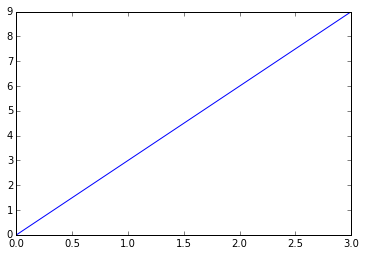In :
plt.plot(x, y, 'o')  # 点图

Out:
[<matplotlib.lines.Line2D at 0x106b32090>]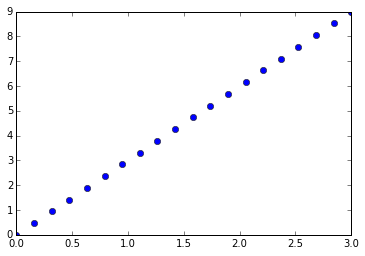• 2D 作图:
In :
image = np.random.rand(30, 30)
plt.imshow(image, cmap=plt.cm.hot)
plt.colorbar()

Out:
<matplotlib.colorbar.Colorbar instance at 0x106a095f0>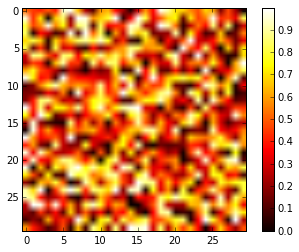#### 1.3.1.5索引和切片¶

In :
a = np.arange(10)
a

Out:
array([0, 1, 2, 3, 4, 5, 6, 7, 8, 9])
In :
a, a, a[-1]

Out:
(0, 2, 9)

In :
a[::-1]

Out:
array([9, 8, 7, 6, 5, 4, 3, 2, 1, 0])

In :
a = np.diag(np.arange(3))
a

Out:
array([[0, 0, 0],
[0, 1, 0],
[0, 0, 2]])
In :
a[1, 1]

Out:
1
In :
a[2, 1] = 10 # 第三行，第二列
a

Out:
array([[ 0,  0,  0],
[ 0,  1,  0],
[ 0, 10,  2]])
In :
a

Out:
array([0, 1, 0])

• 在2D数组中，第一个维度对应行，第二个维度对应列。
• 对于多维度数组 a，a被解释为提取在指定维度的所有元素

In :
a = np.arange(10)
a

Out:
array([0, 1, 2, 3, 4, 5, 6, 7, 8, 9])
In :
a[2:9:3] # [开始:结束:步长]

Out:
array([2, 5, 8])

In :
a[:4]

Out:
array([0, 1, 2, 3])

In :
a[1:3]

Out:
array([1, 2])
In :
a[::2]

Out:
array([0, 2, 4, 6, 8])
In :
a[3:]

Out:
array([3, 4, 5, 6, 7, 8, 9])

Numpy索引和切片的一个小说明...In :
a = np.arange(10)
a[5:] = 10
a

Out:
array([ 0,  1,  2,  3,  4, 10, 10, 10, 10, 10])
In :
b = np.arange(5)
a[5:] = b[::-1]
a

Out:
array([0, 1, 2, 3, 4, 4, 3, 2, 1, 0])

• 试试切片的特色，用起点、结束和步长：从linspace开始，试着从后往前获得奇数，从前往后获得偶数。 重现上面示例中的切片。你需要使用下列表达式创建这个数组：
In :
np.arange(6) + np.arange(0, 51, 10)[:, np.newaxis]

Out:
array([[ 0,  1,  2,  3,  4,  5],
[10, 11, 12, 13, 14, 15],
[20, 21, 22, 23, 24, 25],
[30, 31, 32, 33, 34, 35],
[40, 41, 42, 43, 44, 45],
[50, 51, 52, 53, 54, 55]])

[[1, 1, 1, 1],
[1, 1, 1, 1],
[1, 1, 1, 2],
[1, 6, 1, 1]]

[[0., 0., 0., 0., 0.],
[2., 0., 0., 0., 0.],
[0., 3., 0., 0., 0.],
[0., 0., 4., 0., 0.],
[0., 0., 0., 5., 0.],
[0., 0., 0., 0., 6.]]


[[4, 3, 4, 3, 4, 3],
[2, 1, 2, 1, 2, 1],
[4, 3, 4, 3, 4, 3],
[2, 1, 2, 1, 2, 1]]


### 1.3.1.6 副本和视图¶

In :
a = np.arange(10)
a

Out:
array([0, 1, 2, 3, 4, 5, 6, 7, 8, 9])
In :
b = a[::2]
b

Out:
array([0, 2, 4, 6, 8])
In :
np.may_share_memory(a, b)

Out:
True
In :
b = 12
b

Out:
array([12,  2,  4,  6,  8])
In :
a   # (!)

Out:
array([12,  1,  2,  3,  4,  5,  6,  7,  8,  9])
In :
a = np.arange(10)
c = a[::2].copy()  # 强制复制
c = 12
a

Out:
array([0, 1, 2, 3, 4, 5, 6, 7, 8, 9])
In :
np.may_share_memory(a, c)

Out:
False• 构建一个名为 _prime 形状是 (100,) 的布尔数组，在初始将值都设为True：
In :
is_prime = np.ones((100,), dtype=bool)

• 将不属于素数的0，1去掉
In :
is_prime[:2] = 0


In :
N_max = int(np.sqrt(len(is_prime)))
for j in range(2, N_max):
is_prime[2*j::j] = False

• 看一眼 help(np.nonzero)，然后打印素数
• 接下来:
• 将上面的代码放入名为 prime_sieve.py 的脚本文件
• 运行检查一下时候有效
• 使用埃拉托斯特尼筛法的优化建议
1. 跳过已知不是素数的 j
2. 第一个应该被划掉的数是$j^2$

#### 1.3.1.7象征索引¶

Numpy数组可以用切片索引，也可以用布尔或整形数组（面具）。这个方法也被称为象征索引。它创建一个副本而不是视图。

#### 1.3.1.7.1使用布尔面具¶

In :
np.random.seed(3)
a = np.random.random_integers(0, 20, 15)
a

Out:
array([10,  3,  8,  0, 19, 10, 11,  9, 10,  6,  0, 20, 12,  7, 14])
In :
(a % 3 == 0)

Out:
array([False,  True, False,  True, False, False, False,  True, False,
True,  True, False,  True, False, False], dtype=bool)
In :
mask = (a % 3 == 0)
extract_from_a = a[mask] # 或,  a[a%3==0]
extract_from_a           # 用面具抽取一个子数组

Out:
array([ 3,  0,  9,  6,  0, 12])

In :
a[a % 3 == 0] = -1
a

Out:
array([10, -1,  8, -1, 19, 10, 11, -1, 10, -1, -1, 20, -1,  7, 14])

#### 1.3.1.7.2 用整型数组索引¶

In :
a = np.arange(0, 100, 10)
a

Out:
array([ 0, 10, 20, 30, 40, 50, 60, 70, 80, 90])

In :
a[[2, 3, 2, 4, 2]]  # 注：[2, 3, 2, 4, 2] 是Python列表

Out:
array([20, 30, 20, 40, 20])

In :
a[[9, 7]] = -100
a

Out:
array([   0,   10,   20,   30,   40,   50,   60, -100,   80, -100])

In :
a = np.arange(10)
idx = np.array([[3, 4], [9, 7]])
idx.shape

Out:
(2, 2)
In :
a[idx]

Out:
array([[3, 4],
[9, 7]])

• 同样，重新生成上图中所示的象征索引
• 用左侧的象征索引和右侧的数组创建在为一个数组赋值，例如，设置上图数组的一部分为0。

## 1.3.2 数组的数值操作¶

### 1.3.2.1 元素级操作¶

#### 1.3.2.1.1 基础操作¶

In :
a = np.array([1, 2, 3, 4])
a + 1

Out:
array([2, 3, 4, 5])
In :
2**a

Out:
array([ 2,  4,  8, 16])

In :
b = np.ones(4) + 1
a - b

Out:
array([-1.,  0.,  1.,  2.])
In :
a * b

Out:
array([ 2.,  4.,  6.,  8.])
In :
j = np.arange(5)
2**(j + 1) - j

Out:
array([ 2,  3,  6, 13, 28])

In :
a = np.arange(10000)
%timeit a + 1

100000 loops, best of 3: 11 µs per loop

In :
l = range(10000)
%timeit [i+1 for i in l]

1000 loops, best of 3: 560 µs per loop


In :
c = np.ones((3, 3))
c * c                   # 不是矩阵相乘！

Out:
array([[ 1.,  1.,  1.],
[ 1.,  1.,  1.],
[ 1.,  1.,  1.]])

In :
c.dot(c)

Out:
array([[ 3.,  3.,  3.],
[ 3.,  3.,  3.],
[ 3.,  3.,  3.]])

• 试一下元素级别的简单算术操作
• %timeit 比一下他们与纯Python对等物的时间
• 生成：
• [2**0, 2**1, 2**2, 2**3, 2**4]
• a_j = 2^(3*j) - j

#### 1.3.2.1.2其他操作¶

In :
a = np.array([1, 2, 3, 4])
b = np.array([4, 2, 2, 4])
a == b

Out:
array([False,  True, False,  True], dtype=bool)
In :
a > b

Out:
array([False, False,  True, False], dtype=bool)

In :
a = np.array([1, 2, 3, 4])
b = np.array([4, 2, 2, 4])
c = np.array([1, 2, 3, 4])
np.array_equal(a, b)

Out:
False
In :
np.array_equal(a, c)

Out:
True

In :
a = np.array([1, 1, 0, 0], dtype=bool)
b = np.array([1, 0, 1, 0], dtype=bool)
np.logical_or(a, b)

Out:
array([ True,  True,  True, False], dtype=bool)
In :
np.logical_and(a, b)

Out:
array([ True, False, False, False], dtype=bool)
In :
a = np.arange(5)
np.sin(a)

Out:
array([ 0.        ,  0.84147098,  0.90929743,  0.14112001, -0.7568025 ])
In :
np.log(a)

-c:1: RuntimeWarning: divide by zero encountered in log

Out:
array([       -inf,  0.        ,  0.69314718,  1.09861229,  1.38629436])
In :
np.exp(a)

Out:
array([  1.        ,   2.71828183,   7.3890561 ,  20.08553692,  54.59815003])

In :
a = np.arange(4)
a + np.array([1, 2])

---------------------------------------------------------------------------
ValueError                                Traceback (most recent call last)
<ipython-input-74-82c1c1d5b8c1> in <module>()
1 a = np.arange(4)
----> 2 a + np.array([1, 2])

ValueError: operands could not be broadcast together with shapes (4,) (2,) 

In :
a = np.triu(np.ones((3, 3)), 1)   # 看一下 help(np.triu)
a

Out:
array([[ 0.,  1.,  1.],
[ 0.,  0.,  1.],
[ 0.,  0.,  0.]])
In :
a.T

Out:
array([[ 0.,  0.,  0.],
[ 1.,  0.,  0.],
[ 1.,  1.,  0.]])

In :
a += a.T


• 看一下 np.allclose 的帮助，什么时候这很有用？
• 看一下 np.triunp.tril的帮助。

### 1.3.2.2 基础简化¶

#### 1.3.2.2.1 计算求和¶

In :
x = np.array([1, 2, 3, 4])
np.sum(x)

Out:
10
In :
x.sum()

Out:
10In :
x = np.array([[1, 1], [2, 2]])
x

Out:
array([[1, 1],
[2, 2]])
In :
x.sum(axis=0)   # 列 (第一纬度)

Out:
array([3, 3])
In :
x[:, 0].sum(), x[:, 1].sum()

Out:
(3, 3)
In :
x.sum(axis=1)   # 行 (第二纬度)

Out:
array([2, 4])
In :
x[0, :].sum(), x[1, :].sum()

Out:
(2, 4)

In :
x = np.random.rand(2, 2, 2)
x.sum(axis=2)[0, 1]

Out:
1.2671177193964822
In :
x[0, 1, :].sum()

Out:
1.2671177193964822

#### 1.3.2.2.2 其他简化¶

• 以相同方式运作（也可以使用 axis=

In :
x = np.array([1, 3, 2])
x.min()

Out:
1
In :
x.max()

Out:
3
In :
x.argmin()  # 最小值的索引

Out:
0
In :
x.argmax()  # 最大值的索引

Out:
1

In :
np.all([True, True, False])

Out:
False
In :
np.any([True, True, False])

Out:
True

：可以被应用数组对比：

In :
a = np.zeros((100, 100))
np.any(a != 0)

Out:
False
In :
np.all(a == a)

Out:
True
In :
a = np.array([1, 2, 3, 2])
b = np.array([2, 2, 3, 2])
c = np.array([6, 4, 4, 5])
((a <= b) & (b <= c)).all()

Out:
True

In :
x = np.array([1, 2, 3, 1])
y = np.array([[1, 2, 3], [5, 6, 1]])
x.mean()

Out:
1.75
In :
np.median(x)

Out:
1.5
In :
np.median(y, axis=-1) # 最后的坐标轴

Out:
array([ 2.,  5.])
In :
x.std()          # 全体总体的标准差。

Out:
0.82915619758884995

... 以及其他更多（随着你成长最好学习一下）。

• 假定有 sum ，你会期望看到哪些其他的函数？
• sumcumsum 有什么区别？

populations.txt中的数据描述了过去20年加拿大北部野兔和猞猁的数量（以及胡萝卜）。

In :
cat data/populations.txt

# year	hare	lynx	carrot
1900	30e3	4e3	48300
1901	47.2e3	6.1e3	48200
1902	70.2e3	9.8e3	41500
1903	77.4e3	35.2e3	38200
1904	36.3e3	59.4e3	40600
1905	20.6e3	41.7e3	39800
1906	18.1e3	19e3	38600
1907	21.4e3	13e3	42300
1908	22e3	8.3e3	44500
1909	25.4e3	9.1e3	42100
1910	27.1e3	7.4e3	46000
1911	40.3e3	8e3	46800
1912	57e3	12.3e3	43800
1913	76.6e3	19.5e3	40900
1914	52.3e3	45.7e3	39400
1915	19.5e3	51.1e3	39000
1916	11.2e3	29.7e3	36700
1917	7.6e3	15.8e3	41800
1918	14.6e3	9.7e3	43300
1919	16.2e3	10.1e3	41300
1920	24.7e3	8.6e3	47300


In :
data = np.loadtxt('data/populations.txt')
year, hares, lynxes, carrots = data.T  # 技巧: 将列分配给变量


In :
from matplotlib import pyplot as plt
plt.axes([0.2, 0.1, 0.5, 0.8])
plt.plot(year, hares, year, lynxes, year, carrots)
plt.legend(('Hare', 'Lynx', 'Carrot'), loc=(1.05, 0.5))

Out:
<matplotlib.legend.Legend at 0x1070407d0>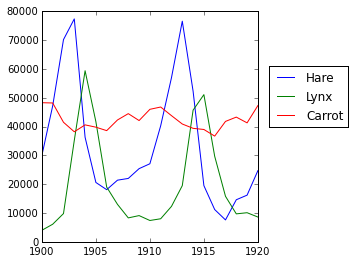In :
populations = data[:, 1:]
populations.mean(axis=0)

Out:
array([ 34080.95238095,  20166.66666667,  42400.        ])

In :
populations.std(axis=0)

Out:
array([ 20897.90645809,  16254.59153691,   3322.50622558])

In :
np.argmax(populations, axis=1)

Out:
array([2, 2, 0, 0, 1, 1, 2, 2, 2, 2, 2, 2, 0, 0, 0, 1, 2, 2, 2, 2, 2])

random_walkIn :
n_stories = 1000 # 行走者的数
t_max = 200      # 我们跟踪行走者的时间


In :
t = np.arange(t_max)
steps = 2 * np.random.random_integers(0, 1, (n_stories, t_max)) - 1
np.unique(steps) # 验证: 所有步长是1或-1

Out:
array([-1,  1])

In :
positions = np.cumsum(steps, axis=1) # axis = 1: 纬度是时间
sq_distance = positions**2


In :
mean_sq_distance = np.mean(sq_distance, axis=0)


In :
plt.figure(figsize=(4, 3))
plt.plot(t, np.sqrt(mean_sq_distance), 'g.', t, np.sqrt(t), 'y-')
plt.xlabel(r"$t$")
plt.ylabel(r"$\sqrt{\langle (\delta x)^2 \rangle}$")

Out:
<matplotlib.text.Text at 0x10b529450>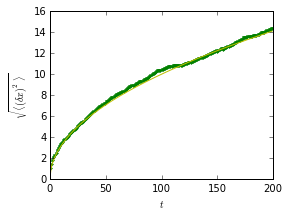### 1.3.2.3 广播¶

• numpy数组的基本操作（相加等）是元素级别的
• 在相同大小的数组上仍然适用。 尽管如此, 也可能在不同大小的数组上进行这个操作，假如Numpy可以将这些数组转化为相同的大小：这种转化称为广播。In :
a = np.tile(np.arange(0, 40, 10), (3, 1)).T
a

Out:
array([[ 0,  0,  0],
[10, 10, 10],
[20, 20, 20],
[30, 30, 30]])
In :
b = np.array([0, 1, 2])
a + b

Out:
array([[ 0,  1,  2],
[10, 11, 12],
[20, 21, 22],
[30, 31, 32]])

In :
a = np.ones((4, 5))
a = 2  # 我们将一个数组的纬度0分配给另一个数组的纬度1
a

Out:
array([[ 2.,  2.,  2.,  2.,  2.],
[ 1.,  1.,  1.,  1.,  1.],
[ 1.,  1.,  1.,  1.,  1.],
[ 1.,  1.,  1.,  1.,  1.]])
In :
a = np.ones((4, 5))
print a
a = 2  # 我们将一个数组的纬度0分配给另一个数组的纬度
a

[ 1.  1.  1.  1.  1.]

Out:
array([[ 2.,  2.,  2.,  2.,  2.],
[ 1.,  1.,  1.,  1.,  1.],
[ 1.,  1.,  1.,  1.,  1.],
[ 1.,  1.,  1.,  1.,  1.]])

In :
a = np.arange(0, 40, 10)
a.shape

Out:
(4,)
In :
a = a[:, np.newaxis]  # 添加一个新的轴 -> 2D 数组
a.shape

Out:
(4, 1)
In :
a

Out:
array([[ 0],
,
,
])
In :
a + b

Out:
array([[ 0,  1,  2],
[10, 11, 12],
[20, 21, 22],
[30, 31, 32]])

In :
mileposts = np.array([0, 198, 303, 736, 871, 1175, 1475, 1544, 1913, 2448])
distance_array = np.abs(mileposts - mileposts[:, np.newaxis])
distance_array

Out:
array([[   0,  198,  303,  736,  871, 1175, 1475, 1544, 1913, 2448],
[ 198,    0,  105,  538,  673,  977, 1277, 1346, 1715, 2250],
[ 303,  105,    0,  433,  568,  872, 1172, 1241, 1610, 2145],
[ 736,  538,  433,    0,  135,  439,  739,  808, 1177, 1712],
[ 871,  673,  568,  135,    0,  304,  604,  673, 1042, 1577],
[1175,  977,  872,  439,  304,    0,  300,  369,  738, 1273],
[1475, 1277, 1172,  739,  604,  300,    0,   69,  438,  973],
[1544, 1346, 1241,  808,  673,  369,   69,    0,  369,  904],
[1913, 1715, 1610, 1177, 1042,  738,  438,  369,    0,  535],
[2448, 2250, 2145, 1712, 1577, 1273,  973,  904,  535,    0]])In :
x, y = np.arange(5), np.arange(5)[:, np.newaxis]
distance = np.sqrt(x ** 2 + y ** 2)
distance

Out:
array([[ 0.        ,  1.        ,  2.        ,  3.        ,  4.        ],
[ 1.        ,  1.41421356,  2.23606798,  3.16227766,  4.12310563],
[ 2.        ,  2.23606798,  2.82842712,  3.60555128,  4.47213595],
[ 3.        ,  3.16227766,  3.60555128,  4.24264069,  5.        ],
[ 4.        ,  4.12310563,  4.47213595,  5.        ,  5.65685425]])

In :
plt.pcolor(distance)
plt.colorbar()

Out:
<matplotlib.colorbar.Colorbar instance at 0x10d8d7170>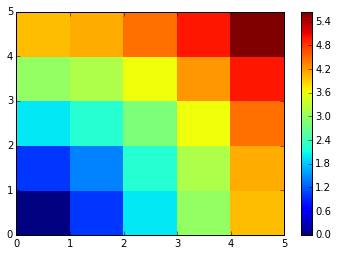In :
x, y = np.ogrid[0:5, 0:5]
x, y

Out:
(array([,
,
,
,
]), array([[0, 1, 2, 3, 4]]))
In :
x.shape, y.shape

Out:
((5, 1), (1, 5))
In :
distance = np.sqrt(x ** 2 + y ** 2)


In :
x, y = np.mgrid[0:4, 0:4]
x

Out:
array([[0, 0, 0, 0],
[1, 1, 1, 1],
[2, 2, 2, 2],
[3, 3, 3, 3]])
In :
y

Out:
array([[0, 1, 2, 3],
[0, 1, 2, 3],
[0, 1, 2, 3],
[0, 1, 2, 3]])

### 1.3.2.4数组形状操作¶

#### 1.3.2.4.1 扁平¶

In :
a = np.array([[1, 2, 3], [4, 5, 6]])
a.ravel()

Out:
array([1, 2, 3, 4, 5, 6])
In :
a.T

Out:
array([[1, 4],
[2, 5],
[3, 6]])
In :
a.T.ravel()

Out:
array([1, 4, 2, 5, 3, 6])

#### 1.3.2.4.2 重排¶

In :
a.shape

Out:
(2, 3)
In :
b = a.ravel()
b = b.reshape((2, 3))
b

Out:
array([[1, 2, 3],
[4, 5, 6]])

In :
a.reshape((2, -1))    # 不确定的值（-1）将被推导

Out:
array([[1, 2, 3],
[4, 5, 6]])

In :
b[0, 0] = 99
a

Out:
array([[99,  2,  3],
[ 4,  5,  6]])

In :
a = np.zeros((3, 2))
b = a.T.reshape(3*2)
b = 9
a

Out:
array([[ 0.,  0.],
[ 0.,  0.],
[ 0.,  0.]])

#### 1.3.2.4.3 添加纬度¶

np.newaxis对象进行索引可以为一个数组添加轴（在上面关于广播的部分你已经看到过了）：

In :
z = np.array([1, 2, 3])
z

Out:
array([1, 2, 3])
In :
z[:, np.newaxis]

Out:
array([,
,
])
In :
z[np.newaxis, :]

Out:
array([[1, 2, 3]])

#### 1.3.2.4.4 纬度重组¶

In :
a = np.arange(4*3*2).reshape(4, 3, 2)
a.shape

Out:
(4, 3, 2)
In :
a[0, 2, 1]

Out:
5
In :
b = a.transpose(1, 2, 0)
b.shape

Out:
(3, 2, 4)
In :
b[2, 1, 0]

Out:
5

In :
b[2, 1, 0] = -1
a[0, 2, 1]

Out:
-1

#### 1.3.2.4.5 改变大小¶

In :
a = np.arange(4)
a.resize((8,))
a

Out:
array([0, 1, 2, 3, 0, 0, 0, 0])

In :
b = a
a.resize((4,))

---------------------------------------------------------------------------
ValueError                                Traceback (most recent call last)
<ipython-input-168-59edd3107605> in <module>()
1 b = a
----> 2 a.resize((4,))

ValueError: cannot resize an array that references or is referenced
by another array in this way.  Use the resize function

• 看一下 reshape 的文档字符串，特别要注意其中关于副本和视图的内容。
• flatten 来替换 ravel。有什么区别？ (提示: 试一下哪个返回视图哪个返回副本)
• 试一下用 transpose 来进行纬度变换。

### 1.3.2.5 数据排序¶

In :
a = np.array([[4, 3, 5], [1, 2, 1]])
b = np.sort(a, axis=1)
b

Out:
array([[3, 4, 5],
[1, 1, 2]])

：每行分别排序！

In :
a.sort(axis=1)
a

Out:
array([[3, 4, 5],
[1, 1, 2]])

In :
a = np.array([4, 3, 1, 2])
j = np.argsort(a)
j

Out:
array([2, 3, 1, 0])
In :
a[j]

Out:
array([1, 2, 3, 4])

In :
a = np.array([4, 3, 1, 2])
j_max = np.argmax(a)
j_min = np.argmin(a)
j_max, j_min

Out:
(0, 2)

• 试一下原地和非原地排序
• 试一下用不同的数据类型创建数组并且排序。
• all 或者 array_equal 来检查一下结果。
• 看一下 np.random.shuffle，一种更快创建可排序输入的方式。
• 合并 ravelsortreshape
• 看一下 sortaxis 关键字，重写一下这个练习。

### 1.3.2.6 总结¶

• 了解如何创建数组：arrayarangeoneszeros
• 了解用 array.shape数组的形状，然后使用切片来获得数组的不同视图：array[::2]等。用 reshape改变数组形状或者用 ravel扁平化。
• 获得数组元素的一个子集，和/或用面具修改他们的值ay and/or modify their values with masks
In :
a[a < 0] = 0

• 了解数组上各式各样的操作，比如找到平均数或最大值 (array.max()array.mean())。不需要记住所有东西，但是应该有条件反射去搜索文档 (线上文档, help(), lookfor())!!
• 更高级的用法：掌握用整型数组索引，以及广播。了解更多的Numpy函数以便处理多种数组操作。

## 1.3.3 数据的更多内容¶

### 1.3.3.1 更多的数据类型¶

#### 1.3.3.1.1 投射¶

“更大”的类型在混合类型操作中胜出：

In :
np.array([1, 2, 3]) + 1.5

Out:
array([ 2.5,  3.5,  4.5])

In :
a = np.array([1, 2, 3])
a.dtype

Out:
dtype('int64')
In :
a = 1.9     # <-- 浮点被截取为整数
a

Out:
array([1, 2, 3])

In :
a = np.array([1.7, 1.2, 1.6])
b = a.astype(int)  # <-- 截取整数
b

Out:
array([1, 1, 1])

In :
a = np.array([1.2, 1.5, 1.6, 2.5, 3.5, 4.5])
b = np.around(a)
b                    # 仍然是浮点

Out:
array([ 1.,  2.,  2.,  2.,  4.,  4.])
In :
c = np.around(a).astype(int)
c

Out:
array([1, 2, 2, 2, 4, 4])

#### 1.3.3.1.2 不同数据类型的大小¶

int8 8 bits
int16 16 bits
int32 32 bits (与32位平台的int相同)
int64 64 bits (与64位平台的int相同)
In :
np.array(, dtype=int).dtype

Out:
dtype('int64')
In :
np.iinfo(np.int32).max, 2**31 - 1

Out:
(2147483647, 2147483647)
In :
np.iinfo(np.int64).max, 2**63 - 1

Out:
(9223372036854775807, 9223372036854775807L)

uint8 8 bits
uint16 16 bits
uint32 32 bits
uint64 64 bits
In :
np.iinfo(np.uint32).max, 2**32 - 1

Out:
(4294967295, 4294967295)
In :
np.iinfo(np.uint64).max, 2**64 - 1

Out:
(18446744073709551615L, 18446744073709551615L)

float16 16 bits
float32 32 bits
float64 64 bits (与浮点相同)
float96 96 bits, 平台依赖 (与 np.longdouble 相同)
float128 128 bits, 平台依赖 (与 np.longdouble相同)
In :
np.finfo(np.float32).eps

Out:
1.1920929e-07
In :
np.finfo(np.float64).eps

Out:
2.2204460492503131e-16
In :
np.float32(1e-8) + np.float32(1) == 1

Out:
True
In :
np.float64(1e-8) + np.float64(1) == 1

Out:
False

complex64 两个 32-bit 浮点
complex128 两个 64-bit 浮点
complex192 两个 96-bit 浮点, 平台依赖
complex256 两个 128-bit 浮点, 平台依赖

• 一半的内存和硬盘大小
• 需要一半的宽带（可能在一些操作中更快）
In :
a = np.zeros((1e6,), dtype=np.float64)
b = np.zeros((1e6,), dtype=np.float32)
%timeit a*a

1000 loops, best of 3: 1.41 ms per loop

In :
%timeit b*b

1000 loops, best of 3: 739 µs per loop

• 但是更大的四舍五入误差 - 有时在一些令人惊喜的地方（即，不要使用它们除非你真的需要）

### 1.3.3.2 结构化的数据类型¶

sensor_code (4个字母的字符)
position (浮点)
value (浮点)
In :
samples = np.zeros((6,), dtype=[('sensor_code', 'S4'),('position', float), ('value', float)])
samples.ndim

Out:
1
In :
samples.shape

Out:
(6,)
In :
samples.dtype.names

Out:
('sensor_code', 'position', 'value')
In :
samples[:] = [('ALFA',   1, 0.37), ('BETA', 1, 0.11), ('TAU', 1,   0.13),('ALFA', 1.5, 0.37), ('ALFA', 3, 0.11),
('TAU', 1.2, 0.13)]
samples

Out:
array([('ALFA', 1.0, 0.37), ('BETA', 1.0, 0.11), ('TAU', 1.0, 0.13),
('ALFA', 1.5, 0.37), ('ALFA', 3.0, 0.11), ('TAU', 1.2, 0.13)],
dtype=[('sensor_code', 'S4'), ('position', '<f8'), ('value', '<f8')])

In :
samples['sensor_code']

Out:
array(['ALFA', 'BETA', 'TAU', 'ALFA', 'ALFA', 'TAU'],
dtype='|S4')
In :
samples['value']

Out:
array([ 0.37,  0.11,  0.13,  0.37,  0.11,  0.13])
In :
samples

Out:
('ALFA', 1.0, 0.37)
In :
samples['sensor_code'] = 'TAU'
samples

Out:
('TAU', 1.0, 0.37)

In :
samples[['position', 'value']]

Out:
array([(1.0, 0.37), (1.0, 0.11), (1.0, 0.13), (1.5, 0.37), (3.0, 0.11),
(1.2, 0.13)],
dtype=[('position', '<f8'), ('value', '<f8')])

In :
samples[samples['sensor_code'] == 'ALFA']

Out:
array([('ALFA', 1.5, 0.37), ('ALFA', 3.0, 0.11)],
dtype=[('sensor_code', 'S4'), ('position', '<f8'), ('value', '<f8')])

：构建结构化数组有需要其他的语言，见这里这里

### 1.3.3.3 面具数组（maskedarray）: 处理缺失值（的传播）¶

• 对于浮点不能用NaN，但是面具对所有类型都适用：
In :
x = np.ma.array([1, 2, 3, 4], mask=[0, 1, 0, 1])
x

Out:
masked_array(data = [1 -- 3 --],
mask = [False  True False  True],
fill_value = 999999)
In :
y = np.ma.array([1, 2, 3, 4], mask=[0, 1, 1, 1])
x + y

Out:
masked_array(data = [2 -- -- --],
mask = [False  True  True  True],
fill_value = 999999)
• 通用函数的面具版本：
In :
np.ma.sqrt([1, -1, 2, -2])

Out:
masked_array(data = [1.0 -- 1.4142135623730951 --],
mask = [False  True False  True],
fill_value = 1e+20)

：有许多其他数组的兄弟姐妹

• 明确的变量名（不需要备注去解释变量里是什么）
• 风格：逗号后及＝周围有空格等。

Python代码风格指南文档字符串惯例页面中给出了相当数据量如何书写“漂亮代码”的规则（并且，最重要的是，与其他人使用相同的惯例!）。

• 除非在一些极特殊的情况下，变量名及备注用英文。

## 1.3.4 高级操作¶

1.3.4.1. 多项式

Numpy也包含不同基的多项式：

In :
p = np.poly1d([3, 2, -1])
p(0)

Out:
-1
In :
p.roots

Out:
array([-1.        ,  0.33333333])
In :
p.order

Out:
2
In :
x = np.linspace(0, 1, 20)
y = np.cos(x) + 0.3*np.random.rand(20)
p = np.poly1d(np.polyfit(x, y, 3))
t = np.linspace(0, 1, 200)
plt.plot(x, y, 'o', t, p(t), '-')

Out:
[<matplotlib.lines.Line2D at 0x10f40c2d0>,
<matplotlib.lines.Line2D at 0x10f40c510>]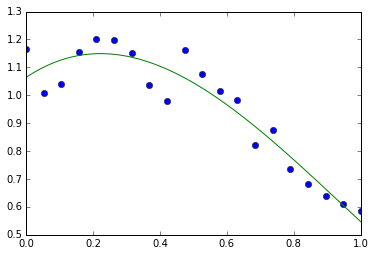#### 1.3.4.1.1 更多多项式（有更多的基）¶

Numpy也有更复杂的多项式接口，支持比如切比雪夫基。

$3x^2 + 2x - 1$:

In :
p = np.polynomial.Polynomial([-1, 2, 3]) # 系数的顺序不同！
p(0)

Out:
-1.0
In :
p.roots()

Out:
array([-1.        ,  0.33333333])
In :
p.degree()  # 在普通的多项式中通常不暴露'order'

Out:
2

In :
x = np.linspace(-1, 1, 2000)
y = np.cos(x) + 0.3*np.random.rand(2000)
p = np.polynomial.Chebyshev.fit(x, y, 90)
t = np.linspace(-1, 1, 200)
plt.plot(x, y, 'r.')
plt.plot(t, p(t), 'k-', lw=3)

Out:
[<matplotlib.lines.Line2D at 0x10f442d10>]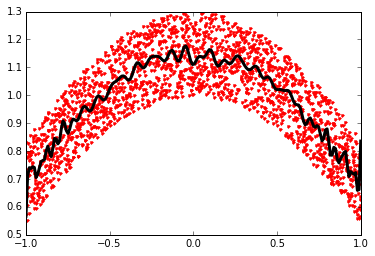### 1.3.4.2 加载数据文件¶

#### 1.3.4.2.1 文本文件¶

# year  hare    lynx    carrot
1900    30e3    4e3     48300
1901    47.2e3  6.1e3   48200
1902    70.2e3  9.8e3   41500
1903    77.4e3  35.2e3  38200
In :
data = np.loadtxt('data/populations.txt')
data

Out:
array([[  1900.,  30000.,   4000.,  48300.],
[  1901.,  47200.,   6100.,  48200.],
[  1902.,  70200.,   9800.,  41500.],
[  1903.,  77400.,  35200.,  38200.],
[  1904.,  36300.,  59400.,  40600.],
[  1905.,  20600.,  41700.,  39800.],
[  1906.,  18100.,  19000.,  38600.],
[  1907.,  21400.,  13000.,  42300.],
[  1908.,  22000.,   8300.,  44500.],
[  1909.,  25400.,   9100.,  42100.],
[  1910.,  27100.,   7400.,  46000.],
[  1911.,  40300.,   8000.,  46800.],
[  1912.,  57000.,  12300.,  43800.],
[  1913.,  76600.,  19500.,  40900.],
[  1914.,  52300.,  45700.,  39400.],
[  1915.,  19500.,  51100.,  39000.],
[  1916.,  11200.,  29700.,  36700.],
[  1917.,   7600.,  15800.,  41800.],
[  1918.,  14600.,   9700.,  43300.],
[  1919.,  16200.,  10100.,  41300.],
[  1920.,  24700.,   8600.,  47300.]])
In :
np.savetxt('pop2.txt', data)
data2 = np.loadtxt('pop2.txt')


：如果你有一个复杂的文本文件，应该尝试：

• np.genfromtxt
• 使用Python的I/O函数和例如正则式来解析（Python特别适合这个工作）

In :
pwd      # 显示当前目录

Out:
u'/Users/cloga/Documents/scipy-lecture-notes_cn'
In :
cd data

/Users/cloga/Documents/scipy-lecture-notes_cn/data

In :
ls

populations.txt


#### 1.3.4.2.2 图像¶

In :
img = plt.imread('data/elephant.png')
img.shape, img.dtype

Out:
((200, 300, 3), dtype('float32'))
In :
plt.imshow(img)

Out:
<matplotlib.image.AxesImage at 0x10fd13f10>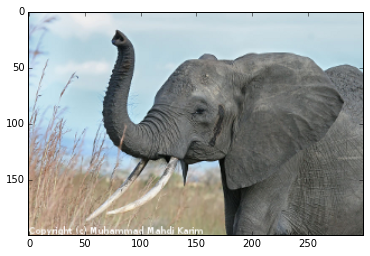In :
plt.savefig('plot.png')
plt.imsave('red_elephant', img[:,:,0], cmap=plt.cm.gray)

<matplotlib.figure.Figure at 0x10fba1750>

In :
plt.imshow(plt.imread('red_elephant.png'))

Out:
<matplotlib.image.AxesImage at 0x11040e150>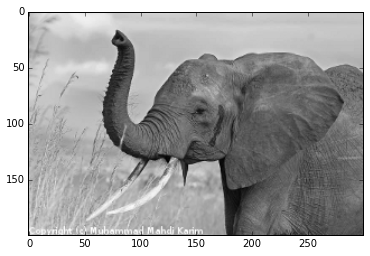In :
from scipy.misc import imsave
imsave('tiny_elephant.png', img[::6,::6])
plt.imshow(plt.imread('tiny_elephant.png'), interpolation='nearest')

Out:
<matplotlib.image.AxesImage at 0x110bfbfd0>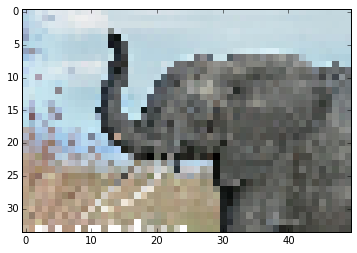#### 1.3.4.2.3 Numpy的自有格式¶

Numpy有自有的二进制格式，没有便携性但是I/O高效：

In :
data = np.ones((3, 3))
np.save('pop.npy', data)
data3 = np.load('pop.npy')


#### 1.3.4.2.4 知名的（并且更复杂的）文件格式¶

• HDF5: h5py, PyTables
• NetCDF: scipy.io.netcdf_file, netcdf4-python, ...
• Matlab: scipy.io.loadmat, scipy.io.savemat
• MatrixMarket: scipy.io.mmread, scipy.io.mmread

... 如果有人使用，那么就可能有一个对应的Python库。

Numpy内部

## 1.3.5 一些练习¶

### 1.3.5.1 数组操作¶

• 从2D数组（不需要显示的输入）:
[[1,  6, 11],
[2,  7, 12],
[3,  8, 13],
[4,  9, 14],
[5, 10, 15]]

• 将数组a的每一列以元素的方式除以数组b (提示: np.newaxis):
In :
a = np.arange(25).reshape(5, 5)
b = np.array([1., 5, 10, 15, 20])

• 难一点的题目：创建10 X 3的随机数数组 （在[0, 1]的范围内）。对于每一行，挑出最接近0.5的数。
• absargsort找到每一行中最接近的列 j
• 使用象征索引抽取数字。（提示：a[i,j]-数组 i 必须包含 j 中成分的对应行数）

### 1.3.5.2 图片操作：给Lena加边框¶

In :
from scipy import misc
lena = misc.lena()


：在旧版的scipy中，你会在 scipy.lena()找到lena。• 让我们用pylab的 imshow函数显示这个图片。
In :
import pylab as plt
lena = misc.lena()
plt.imshow(lena)

Out:
<matplotlib.image.AxesImage at 0x110f51ad0>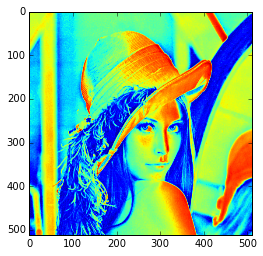• Lena然后以为色彩显示。要将她展示为灰色需要指定一个颜色地图。
In :
plt.imshow(lena, cmap=plt.cm.gray)

Out:
<matplotlib.image.AxesImage at 0x110fb15d0>